# Show Formulas in Excel Instead of the Values

As soon as you type a formula in Excel and hit enter, it would return the calculated result, and the formula would disappear.

That’s how it’s supposed to work.

But what if you want to show formulas in the cells and not the calculated values.

In this Excel tutorial, I will cover the following topics:

• How to Show Formulas in Excel instead of the values.
• How to Print the formulas in Excel.
• How to Show Formulas in Excel in Selected Cells Only.
• What to Do when Excel Shows Formulas Instead of the Calculated Values.

## Show Formulas in Excel Instead of the Values

Here are the steps to show formulas in Excel instead of the value:

• Click on the ‘Formulas’ Tab in the ribbon.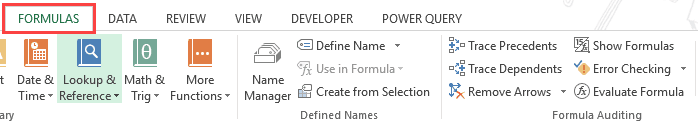• In the Formula Auditing group, click on the Show Formulas option.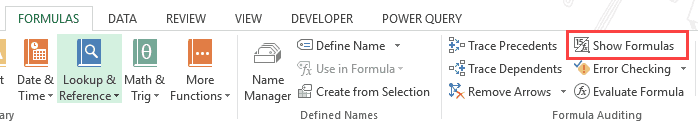As soon as you click on Show Formulas, it will make the formulas in the worksheet visible. It’s a toggle button, so you can click on it again to make the formulas be replaced by its calculated result.

It’s a toggle button, so you can click on it again to make the formulas be replaced by its calculated result.

As shown below, column I has the formulas. As soon as ‘Show Formulas’ button is clicked, the cells show the formulas instead of the value.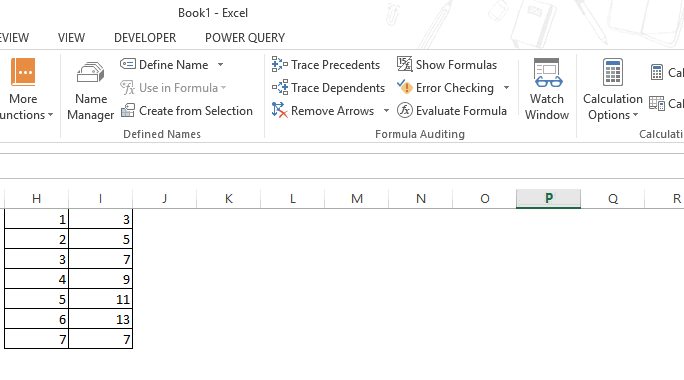You can also use the Excel keyboard shortcut – Control + ` (you will find this key in the top-left part of the keyboard, under the Escape key).

Note: This is a sheet level technique. This means that when you use the Show Formulas option or the shortcut, it will only show the formulas in the active sheet. All the other worksheets will be unaffected. To show formulas in other worksheets, you will have to go to that sheet and use this shortcut (or ribbon button).

In some cases, you may have a lot of worksheets and you want to show the formulas in all the worksheets in the workbook.

Here are the steps that will show the formulas in all the worksheets in Excel:

• Go to the ‘File’ tab. If you’re using Excel 2007, go to Office button.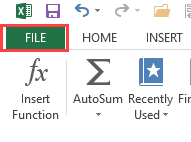• Click on ‘Options’.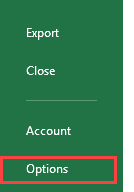• In the left pane, select Advanced.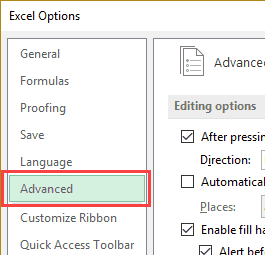• On the right, scroll down to the ‘Display options for this worksheet’ section.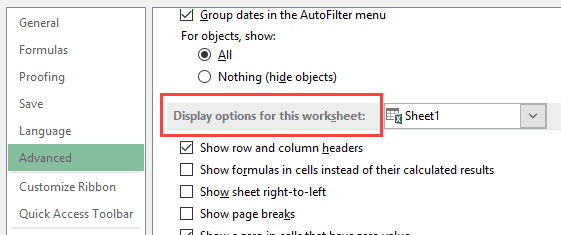• From the drop down, select the worksheet in which you want to show the formulas instead of values.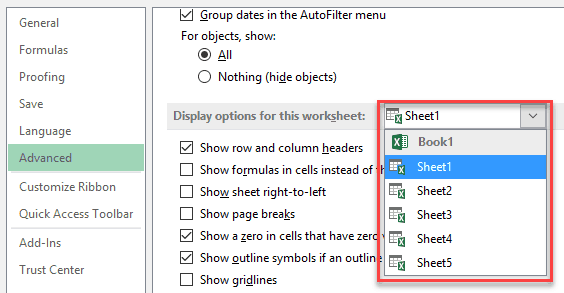• Check the option – ‘Show formulas in cells instead of their calculated results’.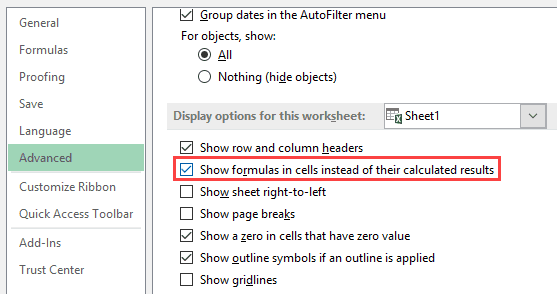• Click OK.

As mentioned, while this may seem to have more steps as compared to a shortcut or the ‘Show Formulas’ button in the ribbon, it’s useful when you have multiple worksheets and you want to show the formulas in all these worksheets.

## How to Print Formulas in Excel

Here are the steps to print formulas in Excel:

• Go to Formula tab.
• Click on the Show Formula option.
• Go to File –> Print.

The above steps would ensure that it prints the formulas and not the values.

## Show Formulas in Excel Instead of the Value in Selected Cells Only

The above methods covered so far would show all the formulas in a worksheet.

However, you may want to show the formulas in some selected cells only.

For example, as an Excel trainer, I often create templates where I show the formula in one cell and its result in another cell (as shown below).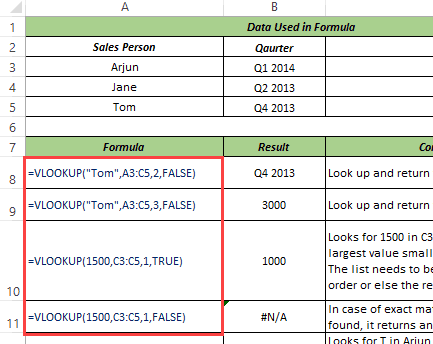Here are the steps to show formulas in Excel in selected cells only:

• Select the cell where you want to show the formula instead of the value.
• Go to Home –> Find & Select –> Replace (keyboard shortcut – Control + H).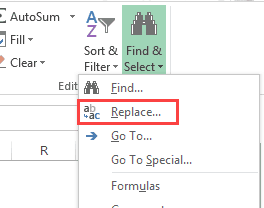• In the Find and Replace dialog box, within the replace tab, enter = in the ‘Find what’ field and ‘= in the ‘Replace with’ field.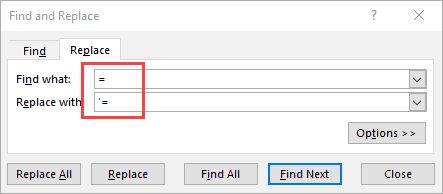• Click on Replace All.

This will show formulas in all the selected cell while the remaining cells would remain unchanged.

Note: Entering a space before the formula makes it a text string and the space character is visible before the equal to sign. On the other hand, using an apostrophe before the equal to sign make the formula a text string, however, the apostrophe isn’t visible in the cell (it shows up only in the formula bar and in the edit mode).

## How to Handle Excel Showing Formulas Instead of Calculated Values

Sometimes, you may find that the cells in Excel are showing the formula instead of the value.

There are a couple of reasons why this may happen:

• The ‘Show Formulas’ mode is enabled or you may have accidently hit the Control + ` shortcut. To disable it, simply use the shortcut again or click on the ‘Show Formula’ option in the Formulas tab.
• It could be due to the presence of a space character or apostrophe before the equal to sign in the formula. The presence of these before the equal to sign makes the cell format as text and the formula shows up instead of the value. To handle this, simply remove these. You can use find and replace to do this.
• If a cell has ‘Text’ formatting applied to it and you enter the formula and hit enter, it will continue to show the formula instead of the calculated value. To fix this issue, go to the Home tab and with the Number group, change the formatting to General.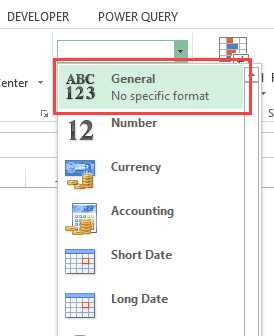You May Also Like the Following Excel Tutorials:FREE EXCEL BOOK

## Get 51 Excel Tips Ebook to skyrocket your productivity and get work done faster

### 3 thoughts on “Show Formulas in Excel Instead of the Values”

1. This doesn’t work. The formulas are still appearing in the cells. Try again

2. Use the following formula
=FORMULATEXT(X17)

It will show the formula in cell X17 in text

3. wonderful tricks